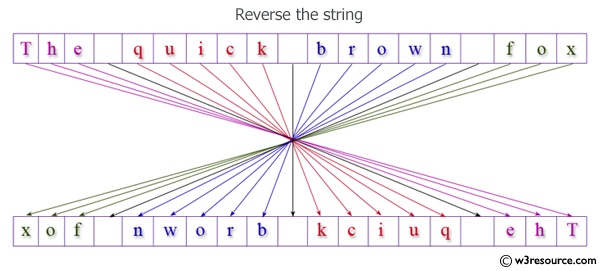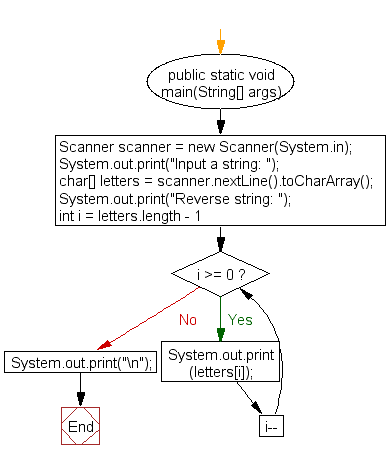﻿ Java - Reverse a string

# Java: Reverse a string

## Java Basic: Exercise-37 with Solution

Write a Java program to reverse a string.

Test Data:
Input a string: The quick brown fox

Pictorial Presentation: Reverse a stringSample Solution:

Java Code:

``````import java.util.Scanner;
public class Exercise37 {
public static void main(String[] args) {
Scanner scanner = new Scanner(System.in);
System.out.print("Input a string: ");
char[] letters = scanner.nextLine().toCharArray();
System.out.print("Reverse string: ");
for (int i = letters.length - 1; i >= 0; i--) {
System.out.print(letters[i]);
}
System.out.print("\n");
}
}
```
```

Sample Output:

```Input a string: The quick brown fox
Reverse string: xof nworb kciuq ehT
```

Flowchart:Java Code Editor:

What is the difficulty level of this exercise?

Test your Programming skills with w3resource's quiz.

﻿

## Java: Tips of the Day

Oddness Check

Is it possible to use this code to accurately determine the odd number?

```public boolean oddOrNot(int num) {
return num % 2 == 1;
}
```

I hope you noticed a trick. If we decide to check a negative odd number in such a way (-5, for example), the remainder of the division will not be equal to one (what does it mean?) Therefore, use a more accurate method:

```public boolean oddOrNot(int num) {
return (num & 1) != 0;
}
```

It not only solves the problem of negative numbers, but also works more productively than its predecessor. Arithmetic and logical operations are performed much faster than multiplication and division.

Ref: https://bit.ly/3giyonA

We are closing our Disqus commenting system for some maintenanace issues. You may write to us at reach[at]yahoo[dot]com or visit us at Facebook